# 一、一维：描述性统计

## 1.1 集中趋势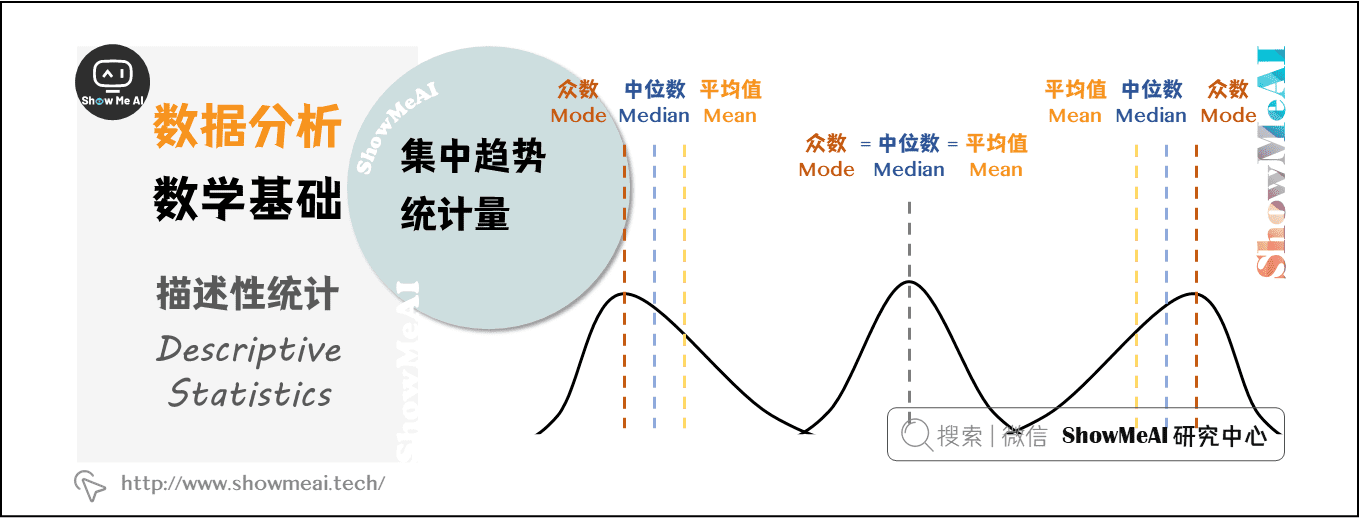## 1.2 离散程度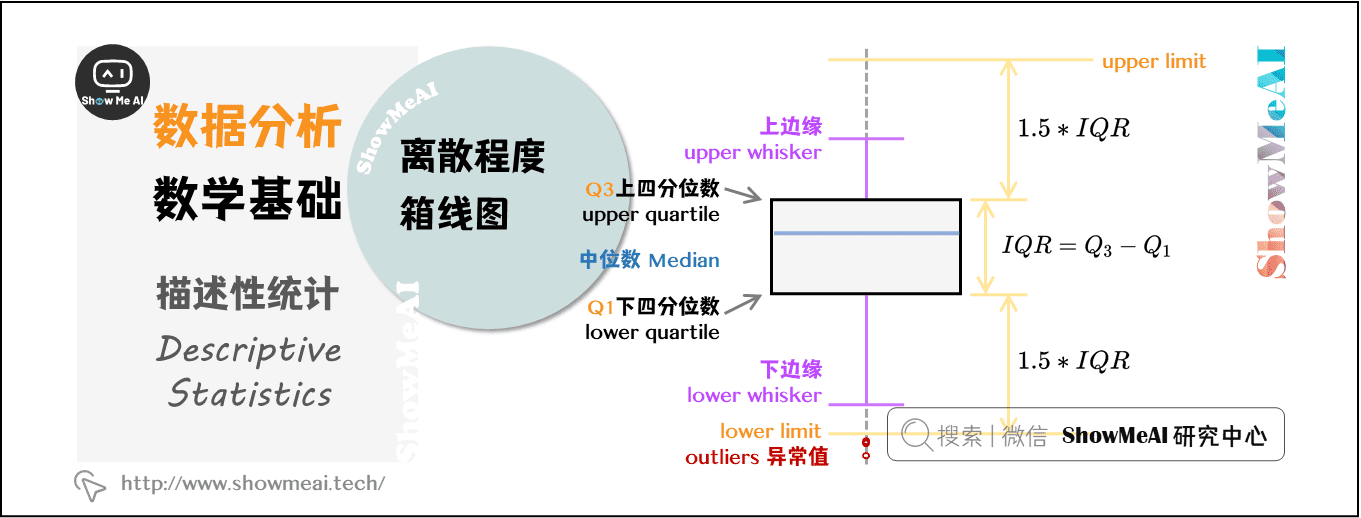### （2）四分位数极差（Inter-Quartile Range， IQR）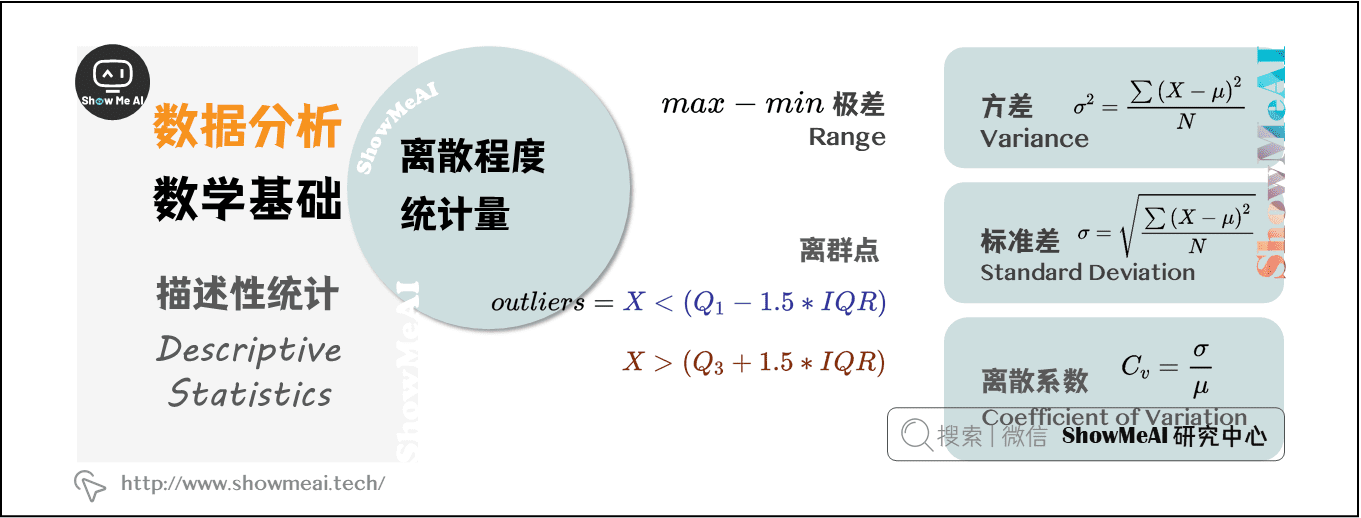### （3）方差（Variance）

σ 2 = ∑ ( X − μ ) 2 N ​​​​ \sigma^{2} = \frac{\sum \left ( X - \mu \right )^{2}}{N} ​​​​

### （4）标准差（Standard Deviation）

σ = ∑ ( X − μ ) 2 N \sigma = \sqrt{\frac{\sum \left ( X - \mu \right )^{2} }{N} }

### （5）离散系数（Coefficient of Variation）

C v = σ μ C_{v} = \frac{\sigma}{\mu}

## 1.3 分布形态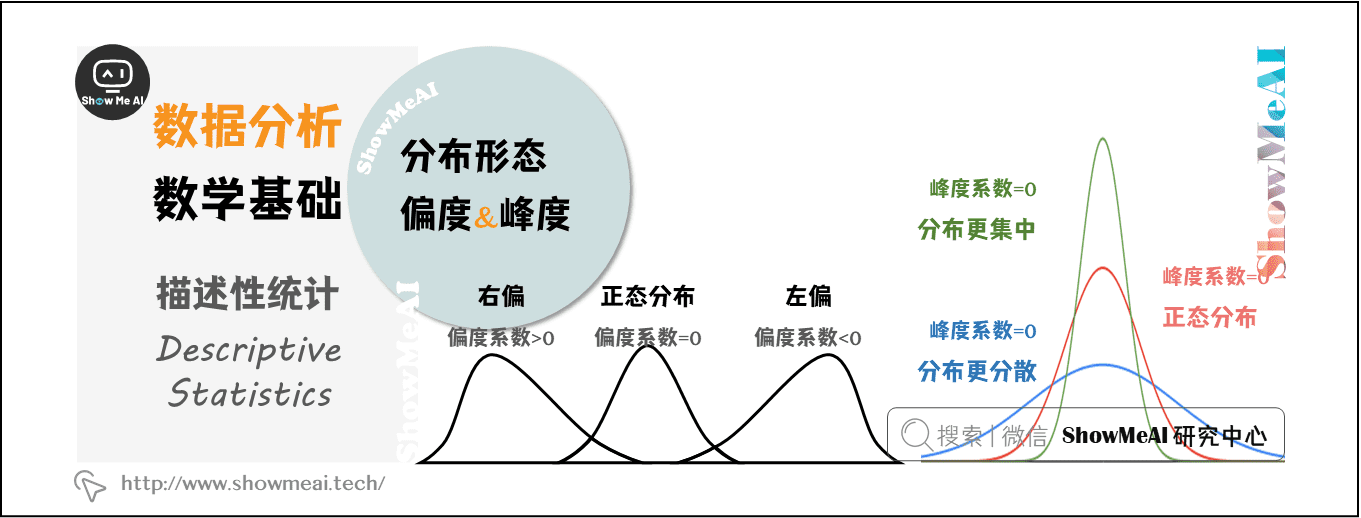### （1）偏度（Skewness）

• 当偏度系数=0时，分布是对称的
• 当偏度系数>0时，分布呈正偏态（右偏）
• 当偏度系数<0时，分布呈负偏态（左偏）

### （2）峰度（Kurtosis）

• 当峰度系数=0时，是正态分布
• 当峰度系数>0时，分布形态陡峭，数据分布更集中
• 当峰度系数<0时，分布形态平缓，数据分布更分散

# 二、交叉维度

## 2.1 相关性和线性回归

### （1）相关系数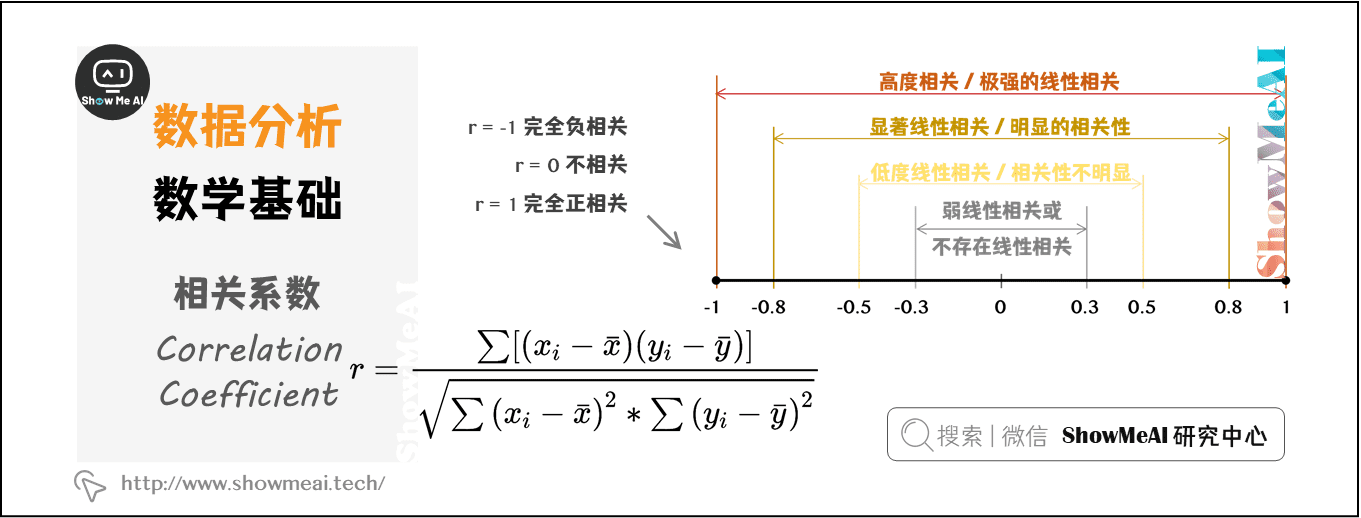### （2）线性回归（Linear Regression）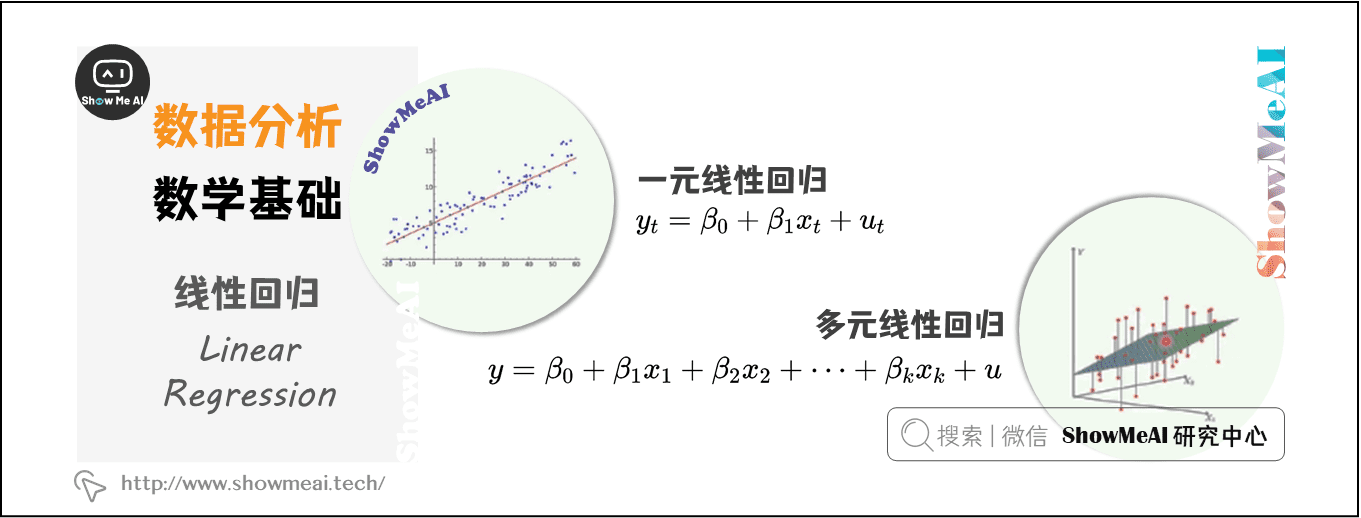# 三、概率论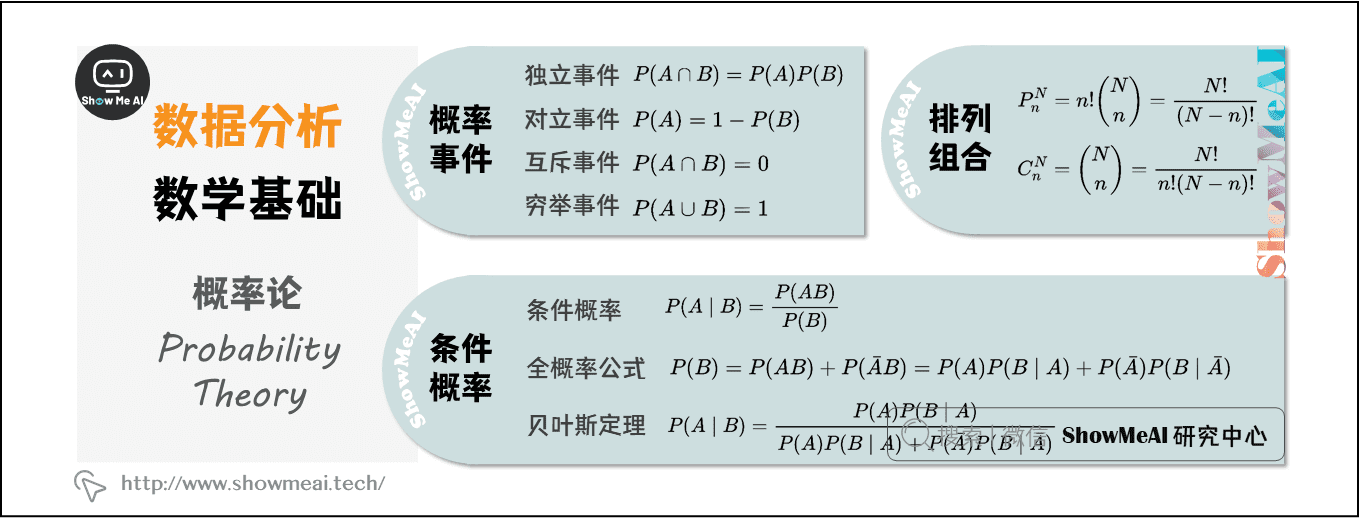## 3.1 概率事件

### （1）独立事件

P ( A ∩ B ) = P ( A ) P ( B ) P\left ( A\cap B \right ) = P(A)P(B)

### （2）对立事件

P ( A ) = 1 − P ( B ) P(A) = 1 - P(B)

### （3）互斥事件

P ( A ∩ B ) = 0 P\left ( A\cap B \right ) = 0

### （4）穷举事件

P ( A ∪ B ) = 1 P\left ( A\cup B \right ) = 1

## 3.2 条件概率

### （1）条件概率

P ( A ∣ B ) = P ( A B ) P ( B ) P(A \mid B) = \frac{P(AB)}{P(B)}

### （2）全概率公式

P ( B ) = P ( A B ) + P ( A ˉ B ) = P ( A ) P ( B ∣ A ) + P ( A ˉ ) P ( B ∣ A ˉ ) P(B) = P(AB) + P(\bar{A} B) = P(A)P(B \mid A) + P(\bar{A} )P(B \mid \bar{A} )

### （3）贝叶斯定理

P ( A ∣ B ) = P ( A ) P ( B ∣ A ) P ( A ) P ( B ∣ A ) + P ( A ˉ ) P ( B ∣ A ˉ ) P(A \mid B) = \frac{ P(A)P(B \mid A) }{ P(A)P(B \mid A) + P(\bar{A})P(B \mid \bar{A}) }

## 3.3 排列组合

### （1）排列

P n N = n ! ( N n ) = N ! ( N − n ) ! P_{n}^{N} = n! \begin{pmatrix} N \\ n \end{pmatrix} = \frac{N!}{ \left (N-n \right )! }

### （2）组合

C n N = ( N n ) = N ! n ! ( N − n ) ! C_{n}^{N} = \begin{pmatrix} N \\ n \end{pmatrix} = \frac{N!}{n! \left (N-n \right )! }

## 3.4 概率分布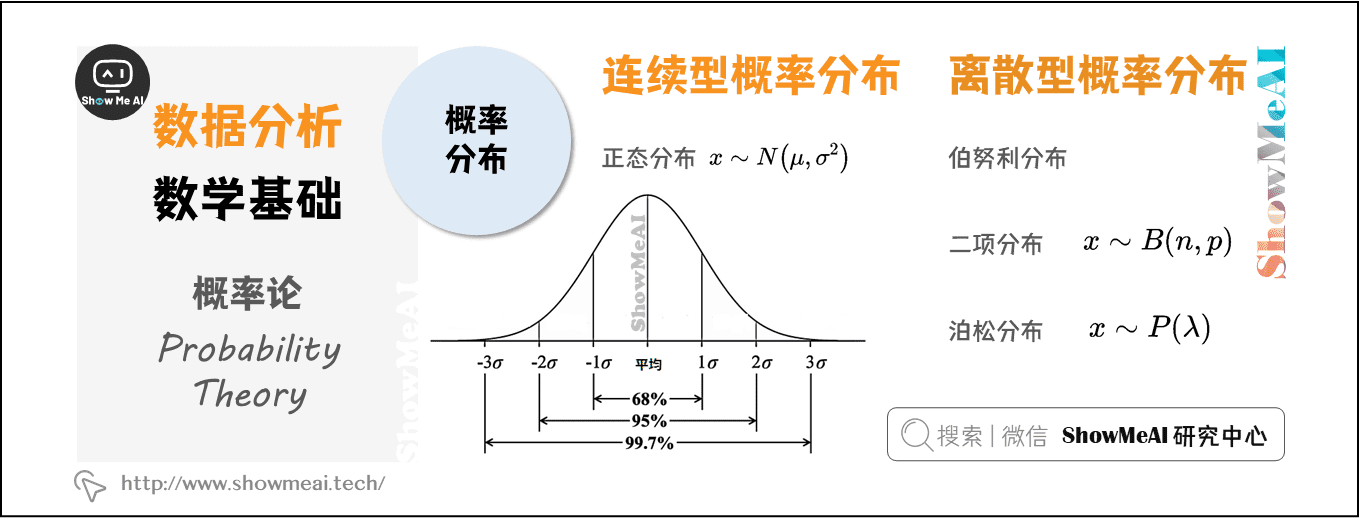### （1）连续型概率分布

x ∼ N ( μ ， σ 2 ) x\sim N\left (\mu ， \sigma^{2} \right)

### （2）离散型概率分布

• 伯努利分布

• 二项分布

n个独立的是/非实验中，成功次数的概率分布。n=1时，二项分布就是伯努利分布

• 泊松分布

# 四、统计推断

## 4.2 置信区间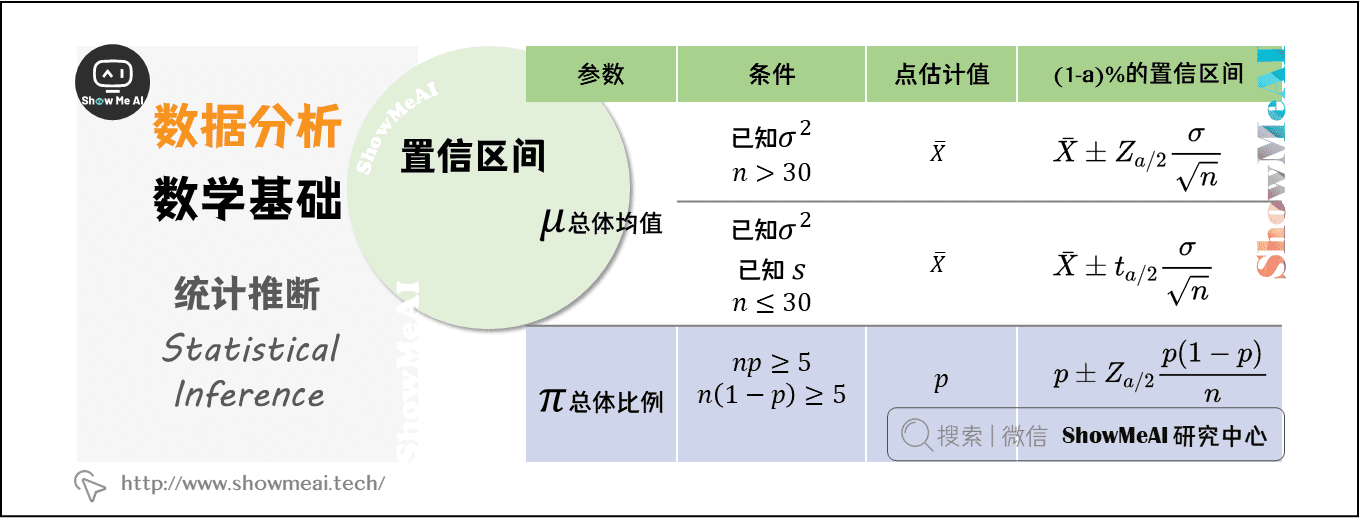## 4.3 假设检验# 下载数据分析速查表

Awesome cheatsheets | ShowMeAI速查表大全 系列包含『编程语言』『AI技能知识』『数据科学工具库』『AI垂直领域工具库』四个板块，追平到工具库当前最新版本，并跑通了所有代码。点击 官网GitHub 获取~👇 数据分析相关速查表（部分）

Python 3速查表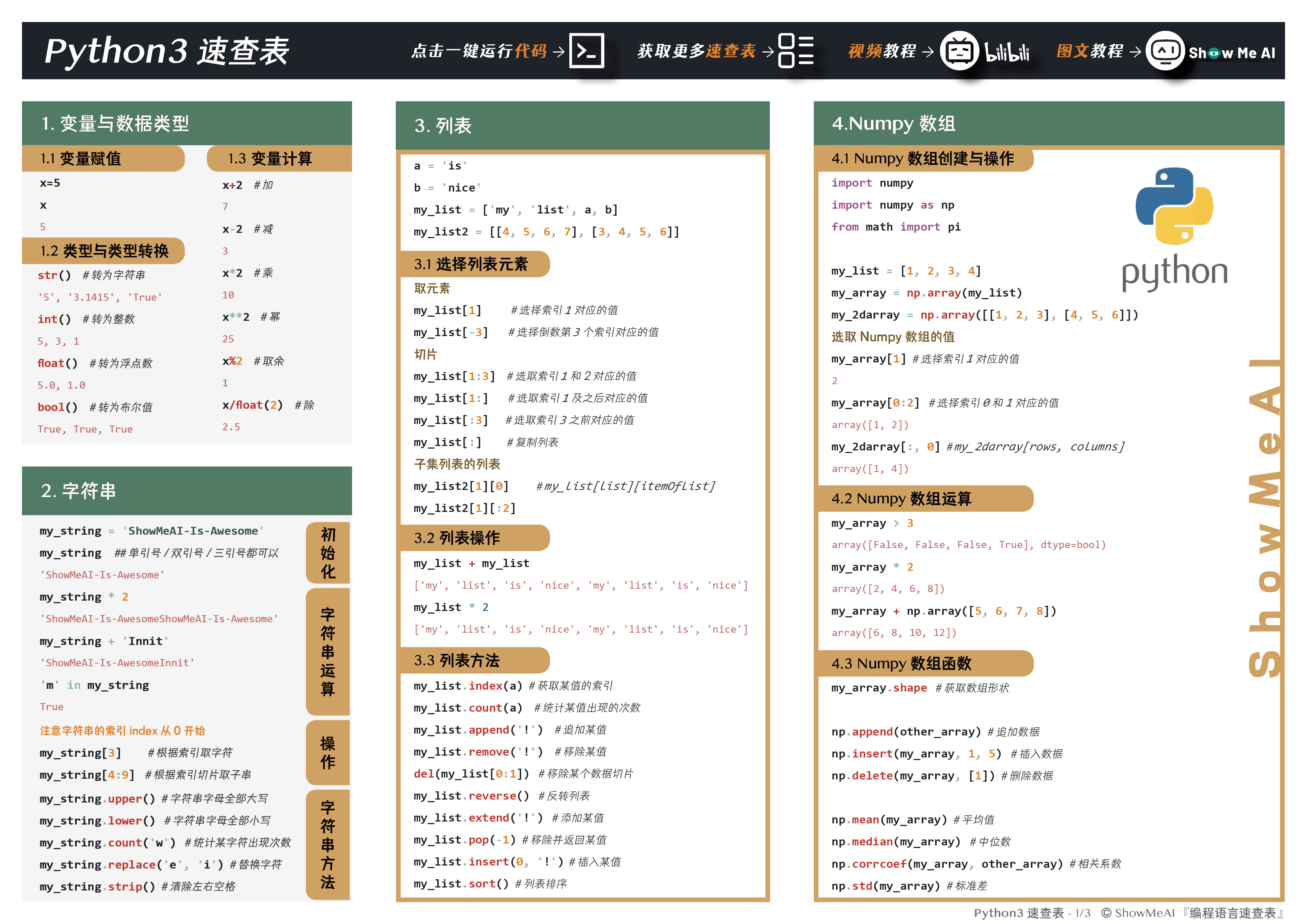一键运行速查表代码 - Python
Numpy 速查表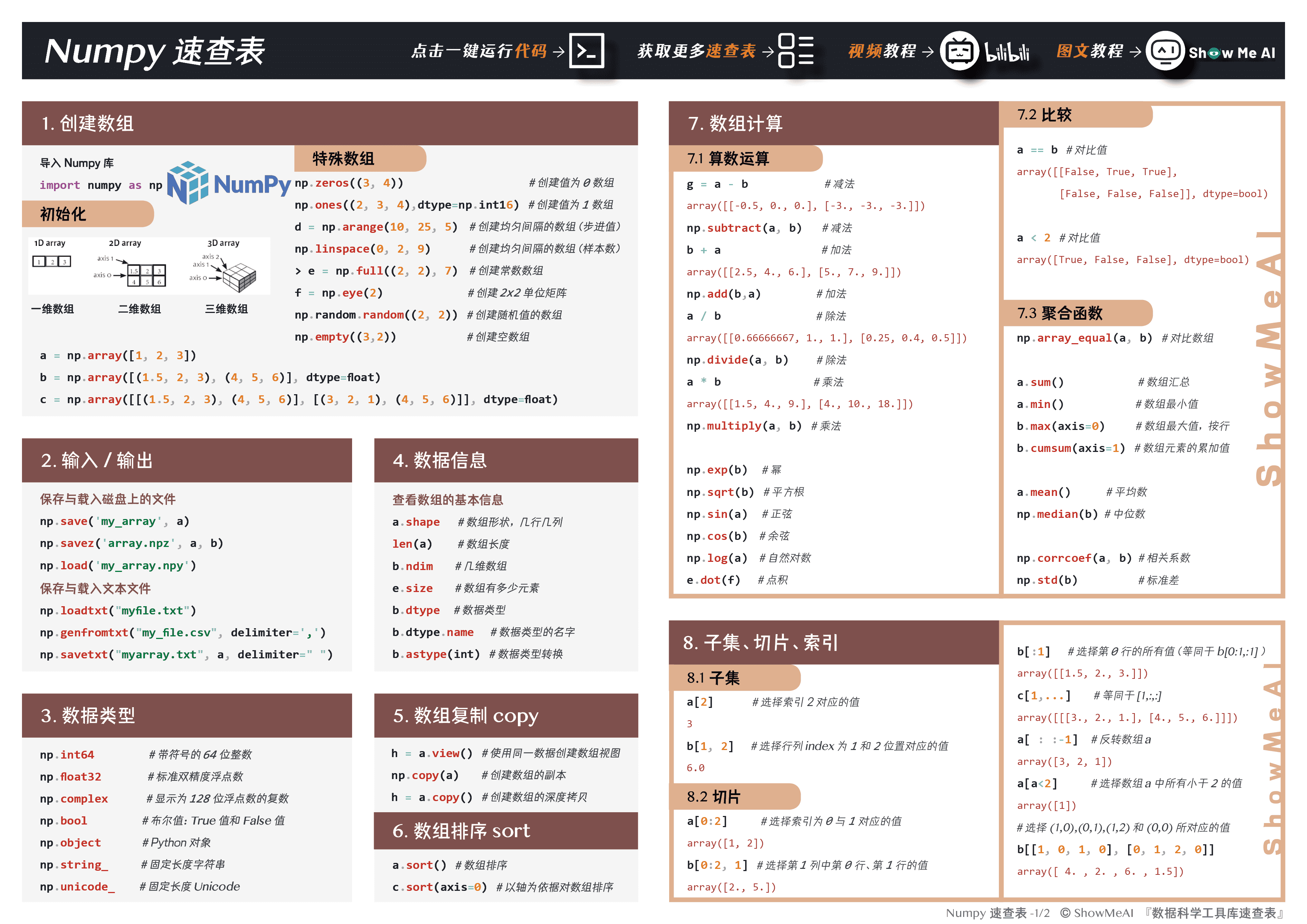一键运行速查表代码 - Numpy
Pandas 速查表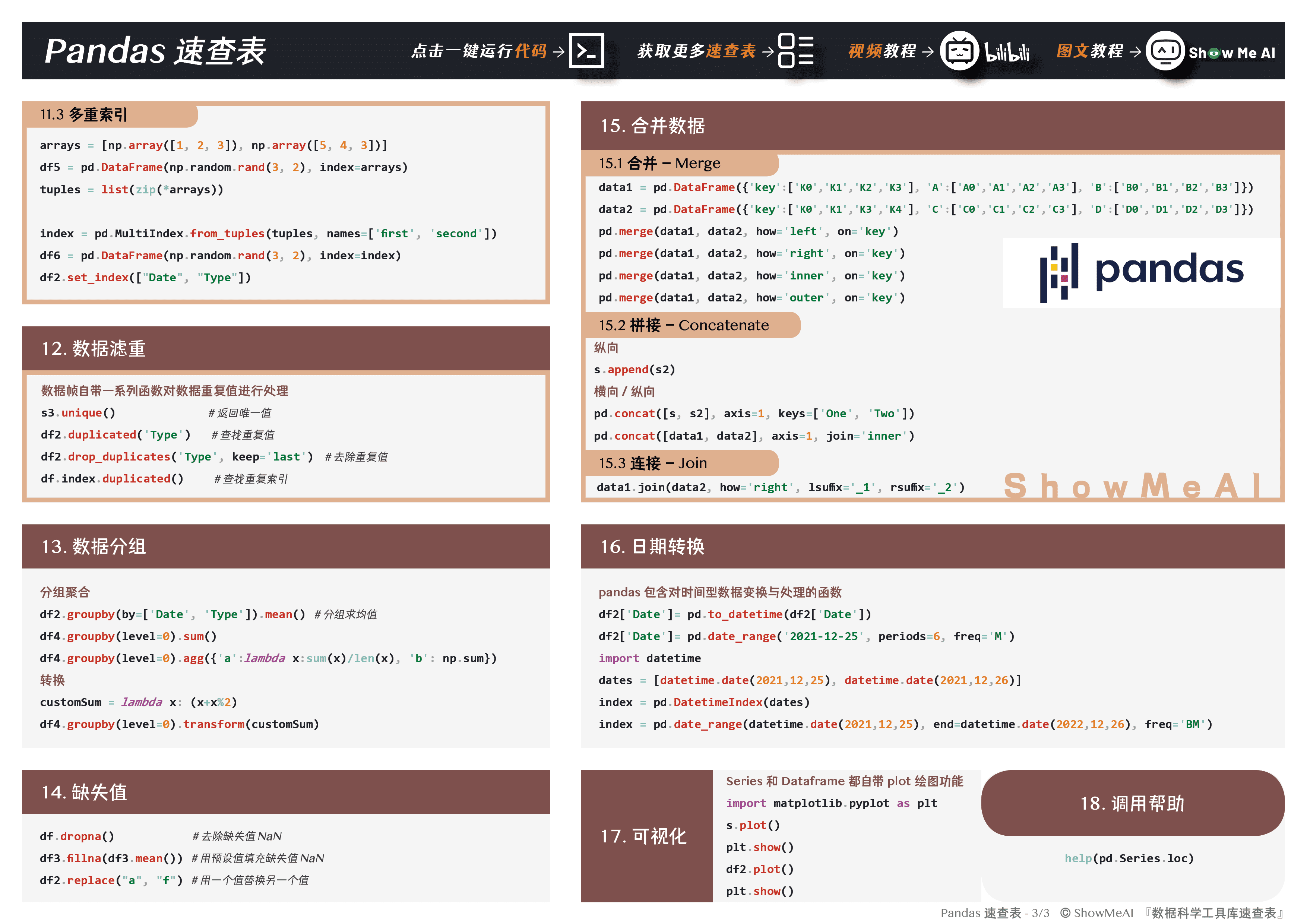一键运行速查表代码 - Pandas
Matplotlib 速查表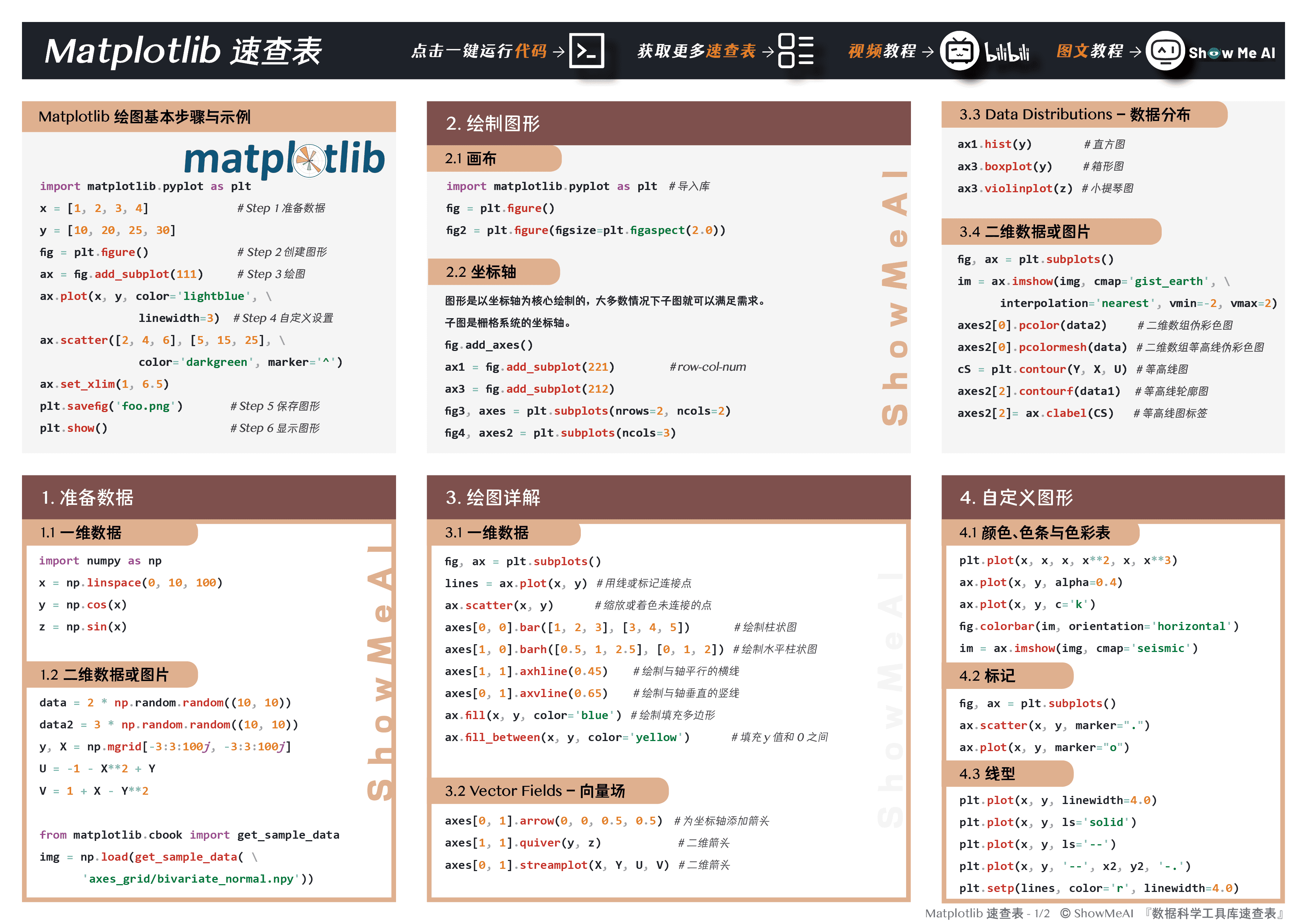一键运行速查表代码 - Matplotlib
Seaborn 速查表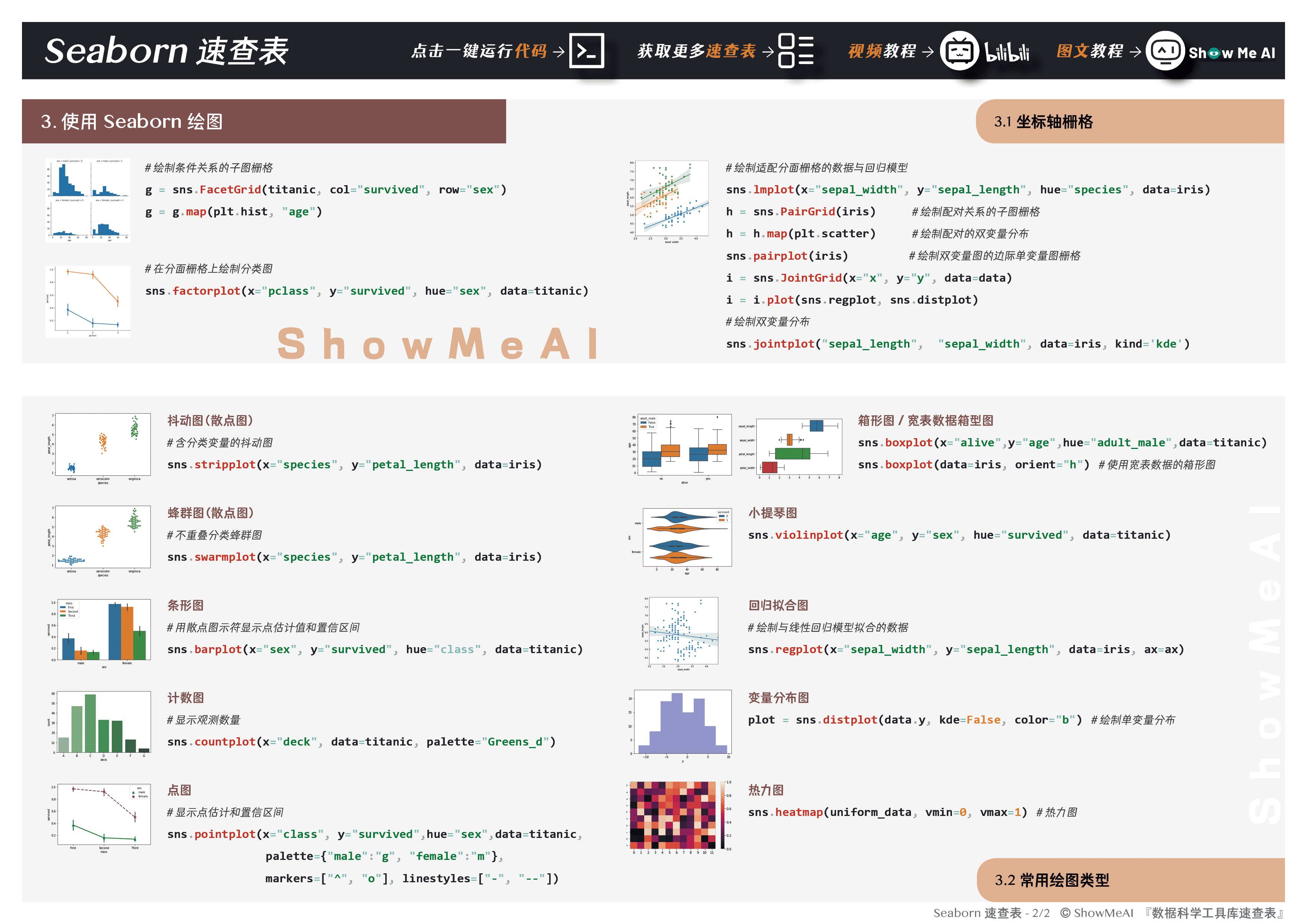一键运行速查表代码 - Seaborn

# ShowMeAI系列教程精选推荐ModelScope旨在打造下一代开源的模型即服务共享平台，为泛AI开发者提供灵活、易用、低成本的一站式模型服务产品，让模型应用更简单！